# Decision Tree Classifier in Python Sklearn with Example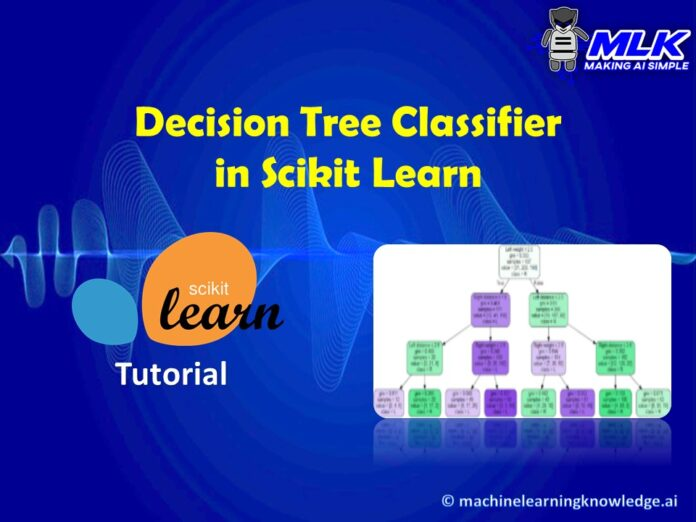In this article, we will go through the tutorial for implementing the Decision Tree in Sklearn (a.k.a Scikit Learn) library of Python. We will first give you a quick overview of what is a decision tree to help you refresh the concept. Then we will implement an end-to-end project with a dataset to show an example of Sklean decision tree classifier with DecisionTreeClassifier() function.

## What is Decision Tree?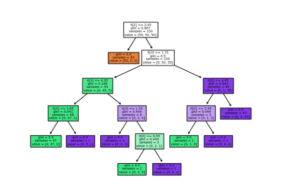Decision tree is a type of supervised learning algorithm that can be used for both regression and classification problems. The algorithm uses training data to create rules that can be represented by a tree structure.

Like any other tree representation, it has a root node, internal nodes, and leaf nodes. The internal node represents condition on attributes, the branches represent the results of the condition and the leaf node represents the class label.

To arrive at the classification, you start at the root node at the top and work your way down to the leaf node by following the if-else style rules. The leaf node where you land up is your class label for your classification problem.
Decision tree can work with both categorical and numerical data. This is in contrast with other machine learning algorithms that cannot work with categorical data and requires encoding to numeric values.

### Making of Decision Tree

For making a decision tree, at each level we have to make a selection of the attributes to be the root node. This is known as attributes selection. This is mainly done using :

• Gini index.
• Information gain.
• chi-square.
How decision trees are created is going to be covered in a later article, because here we are more focused on the implementation of the decision tree in the Sklearn library of Python.

There are some advantages of using a decision tree as listed below –

• The decision tree is a white-box model. We can easily understand any particular condition of the model which results in either true or false.
• It can handle both continuous and categorical data.

Some of the disadvantages of the decision tree are listed below –
• Decision trees may become very large and complex with a large number of attributes.
• A decision tree at times can be sensitive to the training data, a very small variation in data can lead to a completely different tree structure.

## Example of Decision Tree Classifier in Python Sklearn

Scikit Learn library has a module function DecisionTreeClassifier() for implementing decision tree classifier quite easily.

We will show the example of the decision tree classifier in Sklearn by using the Balance-Scale dataset. The goal of this problem is to predict whether the balance scale will tilt to left or right based on the weights on the two sides.

### Importing Libraries

We will start by importing the initial required libraries such as NumPy, pandas, seaborn, and matplotlib.pyplot. The Sklearn modules will be imported in the later section.

In :
```import numpy as np
import pandas as pd
import seaborn as sns
import matplotlib.pyplot as plt
%matplotlib inline```

### Importing Dataset

Next, we import the dataset from the CSV file to the Pandas dataframes.

In :
```col = [ 'Class Name','Left weight','Left distance','Right weight','Right distance']
```
Out:
Class Name Left weight Left distance Right weight Right distance
0 B 1 1 1 1
1 R 1 1 1 2
2 R 1 1 1 3
3 R 1 1 1 4
4 R 1 1 1 5

We can get the overall information of our data set by using the df.info function. From the output, we can see that it has 625 records with 5 fields.

In :
```df.info()
```
[Out] :
```<class 'pandas.core.frame.DataFrame'>
RangeIndex: 625 entries, 0 to 624
Data columns (total 5 columns):
#   Column          Non-Null Count  Dtype
---  ------          --------------  -----
0   Class Name      625 non-null    object
1   Left weight     625 non-null    int64
2   Left distance   625 non-null    int64
3   Right weight    625 non-null    int64
4   Right distance  625 non-null    int64
dtypes: int64(4), object(1)
memory usage: 22.0+ KB```

### Exploratory Data Analysis (EDA)

Let us do a bit of exploratory data analysis to understand our dataset better. We have plotted the classes by using countplot function. We can see in the figure given below that most of the classes names fall under the labels R and L which means Right and Left respectively. Very few data fall under B, which stands for balanced.

In :
```sns.countplot(df['Class Name'])
```
Out: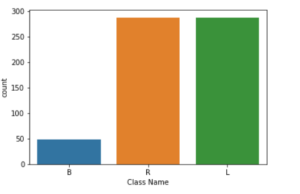In :
```sns.countplot(df['Left weight'],hue=df['Class Name'])
```
Out: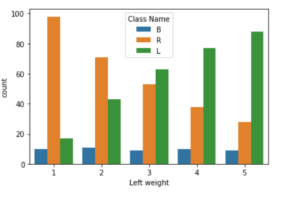In :
```sns.countplot(df['Right weight'],hue=df['Class Name'])
```
Out: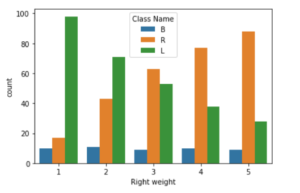### Splitting the Dataset in Train-Test

Before feeding the data into the model we first split it into train and test data using the train_test_split function.

In :
```from sklearn.model_selection import train_test_split
X = df.drop('Class Name',axis=1)
y = df[['Class Name']]
X_train, X_test, y_train, y_test = train_test_split(X, y, test_size = 0.3,random_state=42)
```

### Training the Decision Tree Classifier

We have used the Gini index as our attribute selection method for the training of decision tree classifier with Sklearn function DecisionTreeClassifier().

We have created the decision tree classifier by passing other parameters such as random state, max_depth, and min_sample_leaf to DecisionTreeClassifier().

Finally, we do the training process by using the model.fit() method.

In :
```from sklearn.tree import DecisionTreeClassifier
clf_model = DecisionTreeClassifier(criterion="gini", random_state=42,max_depth=3, min_samples_leaf=5)
clf_model.fit(X_train,y_train)
```
Out:
`DecisionTreeClassifier(max_depth=3, min_samples_leaf=5, random_state=42)`

### Test Accuracy

We will now test accuracy by using the classifier on test data. For this we first use the model.predict function and pass X_test as attributes.

In :
```y_predict = clf_model.predict(X_test)
```

Next, we use accuracy_score function of Sklearn to calculate the accuracty. We can see that we are getting a pretty good accuracy of 78.6% on our test data.

In :
```from sklearn.metrics import accuracy_score,classification_report,confusion_matrix
accuracy_score(y_test,y_predict)
```
Out:
`0.7861276595744681`

### Plotting Decision Tree

We can plot our decision tree with the help of the Graphviz library and passing after a bunch of parameters such as classifier model, target values, and the features name of our data.

In :
```target = list(df['Class Name'].unique())
feature_names = list(X.columns)
```
In :
```from sklearn import tree
import graphviz
dot_data = tree.export_graphviz(clf_model,
out_file=None,
feature_names=feature_names,
class_names=target,
filled=True, rounded=True,
special_characters=True)
graph = graphviz.Source(dot_data)

graph
```
[Out] :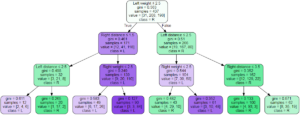We can also get a textual representation of the tree by using the export_tree function from the Sklearn library

In :
```from sklearn.tree import export_text
r = export_text(clf_model, feature_names=feature_names)
print(r)
```
[Out] :
```|--- Left weight <= 2.50
|   |--- Right distance <= 1.50
|   |   |--- Left distance <= 2.50
|   |   |   |--- class: R
|   |   |--- Left distance >  2.50
|   |   |   |--- class: L
|   |--- Right distance >  1.50
|   |   |--- Right weight <= 2.50
|   |   |   |--- class: R
|   |   |--- Right weight >  2.50
|   |   |   |--- class: R
|--- Left weight >  2.50
|   |--- Left distance <= 2.50
|   |   |--- Right weight <= 2.50
|   |   |   |--- class: L
|   |   |--- Right weight >  2.50
|   |   |   |--- class: R
|   |--- Left distance >  2.50
|   |   |--- Right distance <= 3.50
|   |   |   |--- class: L
|   |   |--- Right distance >  3.50
|   |   |   |--- class: L

```

We can save the graph using the save() method.

In :
```graph.save('graph1.jpg')
```
Out:
`'graph1.jpg'`

## Conclusion

Hope you liked our tutorial and now understand how to implement decision tree classifier with Sklearn (Scikit Learn) in Python. We showed you an end-to-end example using a dataset to build a decision tree model for the predictive task using SKlearn DecisionTreeClassifier() function.

1.Merel
•MLK